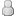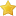• 11 Votes - 4.64 Average
• 1
• 2
• 3
• 4
• 5
12-05-2013, 08:34 PM (This post was last modified: 12-06-2013 10:55 AM by Kokoro.)Post: #117KokoroMemberPosts: 102 Joined: Dec 2013 Reputation: 3
Hi.
Like LunaMoo I was trying to merge two shaders: FXAA with Natural, but I'm not good with this and cant menage Natural shader to work together with FXAA shader. If someone could help me, with what is wrong in my code, and could improve it to work, I would be grateful.

fsh file
Code:
```//================ //Extra options: #define FXAA         1         //ON:1/OFF:0 //================ #define NATURAL      1         //ON:1/OFF:0 //================ #ifdef GL_ES precision mediump float; precision mediump int; #endif uniform sampler2D sampler0; uniform sampler2D sampler1; varying vec4 v_texcoord1; varying vec4 v_texcoord2; varying vec4 v_texcoord3; varying vec4 v_texcoord4; // The inverse of the texture dimensions along X and Y uniform vec2 u_texelDelta; varying vec2 v_texcoord0; //================ void main() {   // The parameters are hardcoded for now, but could be   // made into uniforms to control fromt he program. #if(FXAA==1)   float FXAA_SPAN_MAX = 8.0;   float FXAA_REDUCE_MUL = 1.0/8.0;   float FXAA_REDUCE_MIN = (1.0/128.0);   vec3 rgbNW = texture2D(sampler0, v_texcoord0.xy + (vec2(-1.0, -1.0) * u_texelDelta)).xyz;   vec3 rgbNE = texture2D(sampler0, v_texcoord0.xy + (vec2(+1.0, -1.0) * u_texelDelta)).xyz;   vec3 rgbSW = texture2D(sampler0, v_texcoord0.xy + (vec2(-1.0, +1.0) * u_texelDelta)).xyz;   vec3 rgbSE = texture2D(sampler0, v_texcoord0.xy + (vec2(+1.0, +1.0) * u_texelDelta)).xyz;   vec3 rgbM  = texture2D(sampler0, v_texcoord0.xy).xyz;        vec3 luma = vec3(0.299, 0.587, 0.114);   float lumaNW = dot(rgbNW, luma);   float lumaNE = dot(rgbNE, luma);   float lumaSW = dot(rgbSW, luma);   float lumaSE = dot(rgbSE, luma);   float lumaM  = dot( rgbM, luma);        float lumaMin = min(lumaM, min(min(lumaNW, lumaNE), min(lumaSW, lumaSE)));   float lumaMax = max(lumaM, max(max(lumaNW, lumaNE), max(lumaSW, lumaSE)));        vec2 dir;   dir.x = -((lumaNW + lumaNE) - (lumaSW + lumaSE));   dir.y =  ((lumaNW + lumaSW) - (lumaNE + lumaSE));        float dirReduce = max((lumaNW + lumaNE + lumaSW + lumaSE) * (0.25 * FXAA_REDUCE_MUL), FXAA_REDUCE_MIN);          float rcpDirMin = 1.0/(min(abs(dir.x), abs(dir.y)) + dirReduce);        dir = min(vec2(FXAA_SPAN_MAX,  FXAA_SPAN_MAX),         max(vec2(-FXAA_SPAN_MAX, -FXAA_SPAN_MAX), dir * rcpDirMin)) * u_texelDelta;            vec3 rgbA = (1.0/2.0) * (               texture2D(sampler0, v_texcoord0.xy + dir * (1.0/3.0 - 0.5)).xyz +               texture2D(sampler0, v_texcoord0.xy + dir * (2.0/3.0 - 0.5)).xyz);   vec3 rgbB = rgbA * (1.0/2.0) + (1.0/4.0) * (               texture2D(sampler0, v_texcoord0.xy + dir * (0.0/3.0 - 0.5)).xyz +               texture2D(sampler0, v_texcoord0.xy + dir * (3.0/3.0 - 0.5)).xyz);   float lumaB = dot(rgbB, luma);   if((lumaB < lumaMin) || (lumaB > lumaMax)){     gl_FragColor.xyz=rgbA;   } else {     gl_FragColor.xyz=rgbB;   }   gl_FragColor.a = 1.0; } const mat3 RGBtoYIQ = mat3(0.299, 0.596, 0.212,                            0.587,-0.275,-0.523,                            0.114,-0.321, 0.311); const mat3 YIQtoRGB = mat3(1.0, 1.0, 1.0,                            0.95568806036115671171,-0.27158179694405859326,-1.1081773266826619523,                            0.61985809445637075388,-0.64687381613840131330, 1.7050645599191817149); const vec3 val00 = vec3( 1.2, 1.2, 1.2); void second() { #if(NATURAL==1) vec3 c0,c1; c0 = texture2D(sampler1,v_texcoord1.xy).xyz; c0+=(texture2D(sampler1,v_texcoord1.zy).xyz)*0.25; c0+=(texture2D(sampler1,v_texcoord1.xw).xyz)*0.25; c0+=(texture2D(sampler1,v_texcoord1.zw).xyz)*0.125; c0+= texture2D(sampler1,v_texcoord2.xy).xyz; c0+=(texture2D(sampler1,v_texcoord2.zy).xyz)*0.25; c0+=(texture2D(sampler1,v_texcoord2.xw).xyz)*0.25; c0+=(texture2D(sampler1,v_texcoord2.zw).xyz)*0.125; c0+= texture2D(sampler1,v_texcoord3.xy).xyz; c0+=(texture2D(sampler1,v_texcoord3.zy).xyz)*0.25; c0+=(texture2D(sampler1,v_texcoord3.xw).xyz)*0.25; c0+=(texture2D(sampler1,v_texcoord3.zw).xyz)*0.125; c0+= texture2D(sampler1,v_texcoord4.xy).xyz; c0+=(texture2D(sampler1,v_texcoord4.zy).xyz)*0.25; c0+=(texture2D(sampler1,v_texcoord4.xw).xyz)*0.25; c0+=(texture2D(sampler1,v_texcoord4.zw).xyz)*0.125; c0*=0.153846153846; c1=RGBtoYIQ*c0; c1=vec3(pow(c1.x,val00.x),c1.yz*val00.yz); gl_FragColor.xyz=YIQtoRGB*c1; }```

vsh file
Code:
```uniform vec4 u_texelDelta; attribute vec4 a_position; attribute vec2 a_texcoord0; varying vec2 v_texcoord0; varying vec4 v_texcoord1; varying vec4 v_texcoord2; varying vec4 v_texcoord3; varying vec4 v_texcoord4; void main() {   v_texcoord0 = a_texcoord0;   gl_Position = a_position;   v_texcoord1=a_texcoord0.xyxy+vec4(-0.5,-0.5,-1.5,-1.5)*u_texelDelta.xyxy;   v_texcoord2=a_texcoord0.xyxy+vec4( 0.5,-0.5, 1.5,-1.5)*u_texelDelta.xyxy;   v_texcoord3=a_texcoord0.xyxy+vec4(-0.5, 0.5,-1.5, 1.5)*u_texelDelta.xyxy;   v_texcoord4=a_texcoord0.xyxy+vec4( 0.5, 0.5, 1.5, 1.5)*u_texelDelta.xyxy; }```
 « Next Oldest | Next Newest »